Entering text into the input field will update the search result below

# What Is The Relationship Between Interest Rates, Growth, And Inflation?

Interest rates are on the rise, at their highest levels in over 4 years. What is that telling us, if anything, about growth and inflation? Let's take a look…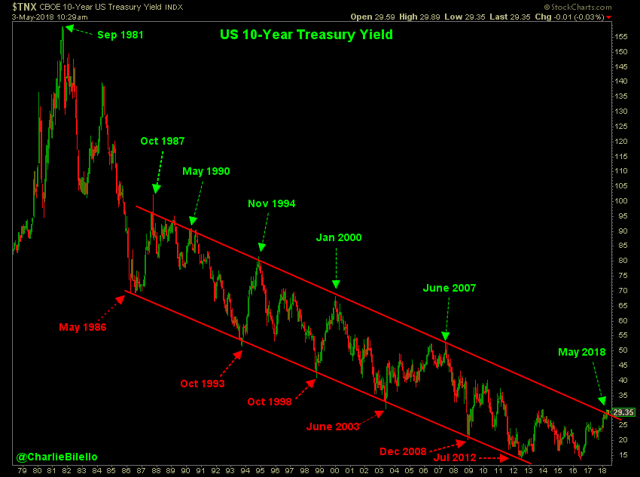While you often hear commentators suggesting there is a strong relationship between bond yields and the real economy, the evidence seems to be lacking. Since 1930, the correlation between annual change in real GDP and the 10-year treasury yield is effectively 0 (-0.05).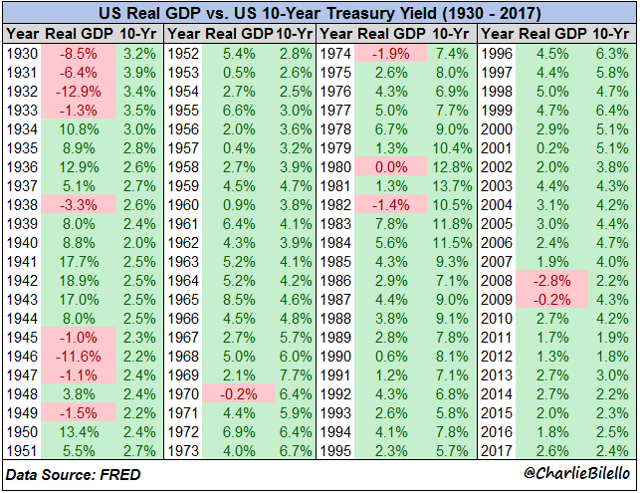There have been years in which interest rates are high and real GDP is low (1970, 1974, 1980, 1982, and 1990) and other years in which interest rates are low and real GDP is high (1936, 1939-1943, and 1950).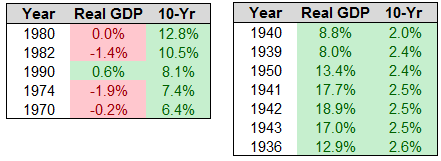Examining quarterly data since 1962, we find a 0.05 correlation between the 10-Year Treasury yield and real GDP. The level of interest rates by itself seems to tell us nothing about real economic growth.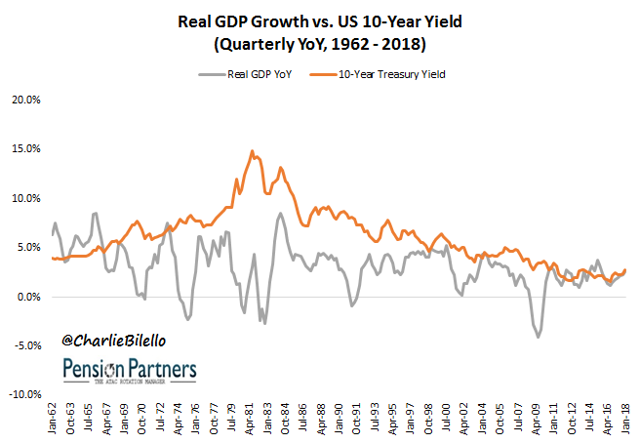Data source for all charts and tables herein: FRED.

But what about nominal GDP growth? There does appear to be some information there, with a correlation of 0.58 between 10-Year yields and nominal GDP. Generally speaking, the higher the level of interest rates, the higher the level of nominal growth and vice versa.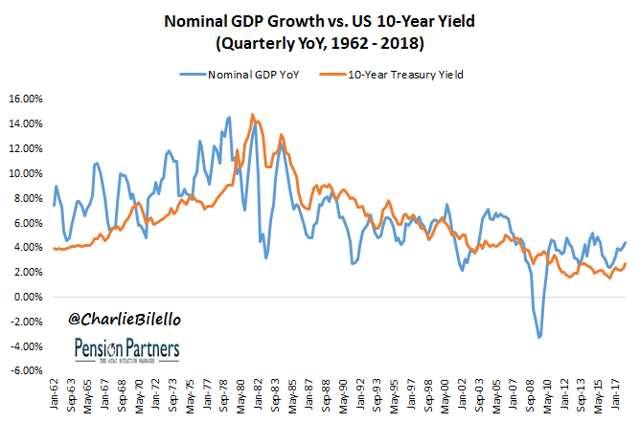As you probably noticed in the chart above, though, swings in nominal GDP tend to be much larger than swings in interest rates. If we smooth out these changes in GDP over a 5-year period, the correlation between the two variables moves up to 0.85. So there appears to be a strong relationship between the average nominal GDP over the past 5 years and the current level of interest rates.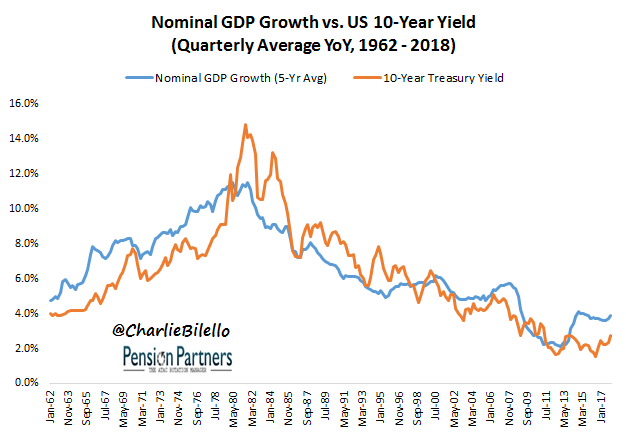Why does a rise in interest rates tend to coincide with a rise in nominal GDP but not necessarily real GDP?

Because of varying changes in inflation, which may rise more (detracting from real